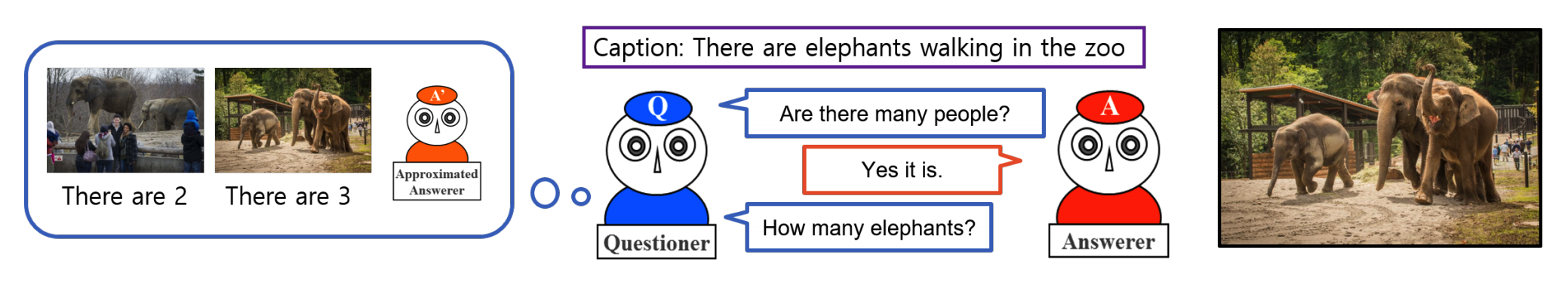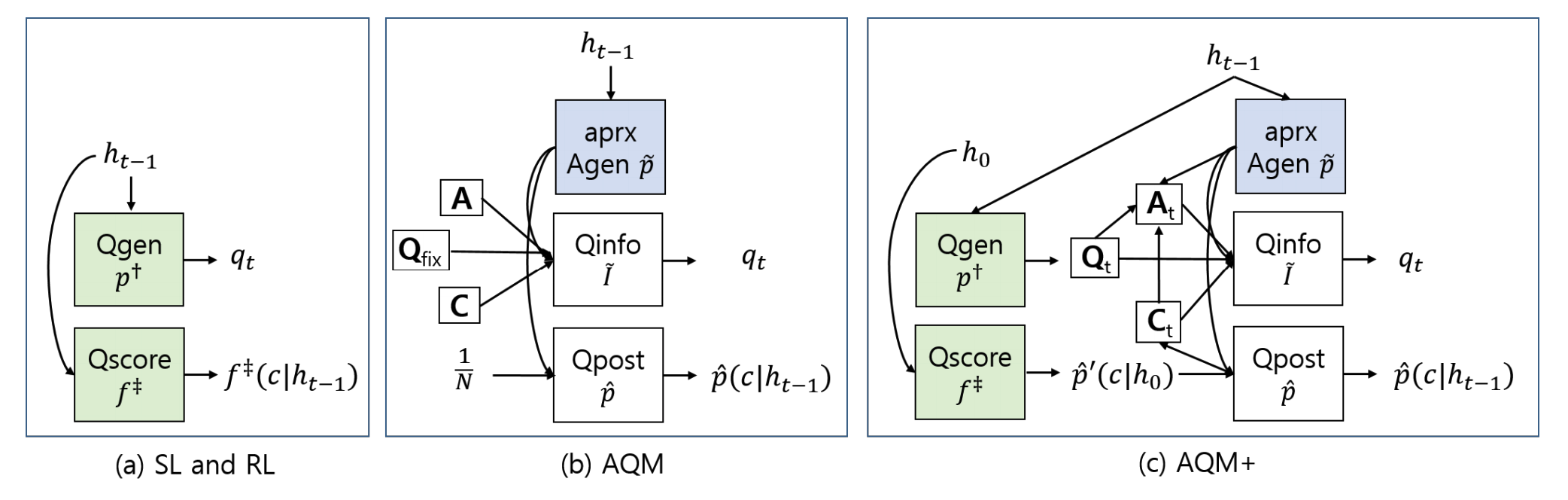# Large-Scale Answerer in Questioner's Mind for Visual Dialog Question Generation

## WHY?

AQM solves visual dialogue tasks with information theoratic approach. However, the information gain by each candidate question needs to be calculated explicitly which leads to lack of scalability. This paper suggests AQM+ to solve large-scale problem.

## WHAT?AQM+ is sampling based approximation to AQM algorithm. AQM+ is different from AQM in 3 ways. First, instead of Q-sampler, candidate questions are sampled using beam search. Second, while the answerer model of AQM was a binary classifier, that of AQM+ is an RNN generator. Third, a portion of answer candidates and labels(top k) are used to approximate information gain.

\tilde{I}_{topk}[C, A_t; q_t, h_{t-1}] = \sum_{a_t\in \mathbf{A}_{t, topk}}
= \sum_{a_t\in \mathbf{A}_{t, topk}(q_t)}\sum_{c\in \mathbf{C}_{t, topk}} \hat{p}_{reg}(c|h_{t-1})\tilde{p}_{reg}(a_t|c, q_t, h_{t-1}) \ln \frac{\tilde{p}_{reg}(a_t|c, q_t, h_{t-1})}{\tilde{p}_{reg}'(a_t|q_t, h_{t-1})}\\
\hat{p}_{reg}(c|h_{t-1}) = \frac{\hat{p}(c|h_{t-1})}{\sum_{c\in \mathbf{C}_{t, topk}}p(c|h_{t-1})}\\
\tilde{p}_{reg}(a_t|c, q_t, h_{t-1}) = \frac{\tilde{p}(a_t|c, q_t, h_{t-1})}{\sum_{a_t\in \mathbf{A}_{t, topk}(q_t)}\tilde{p}(a_t|c, q_t, h_{t-1})}\\
\tilde{p}_{reg}'(a_t|q_t, h_{t-1}) = \sum_{c\in \mathbf{C}_{t, topk}}\hat{p}_{reg}(c|h_{t-1})\cdot\tilde{p}_{reg}(a_t|c, q_t, h_{t-1})

\mathbf{C}_{t, topk}$\mathbf{C}_{t, topk}$ refers to top-K posterior test images from Qpost \hat{p}_{reg}(c\|h_{t-1})$\hat{p}_{reg}(c\|h_{t-1})$. \mathbf{Q}_{t, topk}$\mathbf{Q}_{t, topk}$ refers to top-K likelihood questions using beam search from Qgen p(q_t\|h_{t-1})$p(q_t\|h_{t-1})$. \mathbf{A}_{t, topk}(q_t)$\mathbf{A}_{t, topk}(q_t)$ refers to top-1 generated answers for each question and each class from aprxAgen \tilde{p}(a_t\|c, q_t, h_{t-1})$\tilde{p}(a_t\|c, q_t, h_{t-1})$. Similar to AQM, approximation of the answer generator can be either indA or depA.

## So?Instead of GuessWhat, AQM+ is applied to GuessWhich which is more complicated version of GuessWhat. The key differences of GuessWhich are that the questioner has to guess one image out of 9628 images by asking questions and that the answers of answerer are not limited to binary. Since there are much more labels in this task, analytic computation of information gain is almost intractable in AQM. AQM+ performed better than SL-Q and RL-QA in various settings.

Powered by aiden